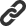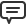# Blog

All Blog Posts  |  Next Post  |  Previous Post

#Realizing symbolic operations with user defined data

Bookmarks:

#### Friday, March 1, 2019

The main functionality of TMS Analytics & Physics developing library is evaluating math formula. The library supports evaluation of expressions containing real and complex numbers, common fractions, 3D vectors and tensors, arrays and matrixes. Nevertheless, one of the advantages of the library is that it can work with data of any types, specific for the developing application. The evaluation engine of the library is abstract and not bounded with real or other data types. Therefore, the library allows easily writing extensions for working with user defined types.

Let us consider the example for simple data type. Suppose we are writing some application and there is 2D vector type:
```type
TVec2D = record
public
X, Y: real;

end;
```
And the application needs functionality for evaluating symbolic formula with vector data types. First, we need to use vector data in math formulae. The simplest way is introducing variables, containing this data type. This can be done using the following code:

```var
translator: TTranslator;

var
x: TVariable;
v: TVec2D;
begin
translator:= TTranslator.Create;

v.X:= 2.0;
v.Y:=-3.0;
x:= TObjectVariable.Create('u', TValue.From<TVec2D>(v), nil);

v.X:= 0.0;
v.Y:= 1.0;
x:= TObjectVariable.Create('v', TValue.From<TVec2D>(v), nil);

v.X:= 1.5;
v.Y:= 2.5;
x:= TObjectVariable.Create('w', TValue.From<TVec2D>(v), nil);
end;
```

The first line of code creates an instance of the TTranslator class, which realizes main functionality for working with math formula. Then we initialized a value of 2D vector and created the instance of TObjectVariable class. This class implements variable that can contain data of any type – TVec2D in the case. Then the variable added to the translator instance using ‘Add’ method. Next, other two variables introduced and now we can use variables with names ‘u’, ‘v’ and ‘w’ in math expressions.

The next step is introducing math operations for vector data. Say we need evaluating sum of vectors ‘u+v’. Then we must define the ‘+’ operator for 2D vectors. Here we suppose that the vector type is entirely realized and it contains the addition method:

```type
TVec2D = record
…

public
class function Add(const v1, v2: TVec2D): TVec2D;
end;

…

class function TVec2D.Add(const v1, v2: TVec2D): TVec2D;
begin
result.X:= v1.X+v2.X;
result.Y:= v1.Y+v2.Y;
end;
```

To introduce an operation for specific data types we must create the descendant for one of the base operator classes. Code for ‘+’ operator is the following:

```type
protected
function TypedOperation(const v1, v2: TVec2D): TVec2D; override;
public
class function IsRealized: boolean; override;
end;

…

function TVectorAdd.TypedOperation(const v1, v2: TVec2D): TVec2D;
begin
end;

begin
result:= true;
end;
```

The class ‘TVectorAdd’ inherited from the base generic class ‘TGenericAddOperator’. This means that it is for defining ‘+’ operation in math formulae. Its generic parameters define that the addition operation is implemented for two vectors and the result type is 2D vector. The main overridden method ‘TypedOperation’ implements the function, which called when math formula contains ‘+’ operation with two vector operands. Another method ‘IsRealized’ returns true and informs the Analytics Evaluation Engine (AEE) that the class is entirely realized. The AEE uses Delphi reflection mechanisms to find the class and automatically applies it when required for formula evaluation.

Now we can write the code for symbolic formula evaluation with 2D vector data. Common code for any symbolic formula evaluation, including syntax checking and error processing, is the following:

```var
f: string;
r: TValue;
begin
f:= EditF.Text;

try
if translator.CheckSyntax(f) then
begin
r:= translator.Calculate(f);

// code for processing result ‘r’
RichEditOut.Text:= RichEditOut.Text +#13#13+f+' = '+TUtilities.SafeToString(r);
end;
except
on ex: Exception do
begin
// code for error (exception) processing
RichEditOut.Text:= RichEditOut.Text +#13#13+f+#13+ex.Message;
end;
end;
end;
```

Where ‘f’ is the symbolic formula for evaluation, ‘r’ is the result value. The AEE uses as the result ‘TValue’ type from RTTI unit, because it can ‘wrap’ data of any type.

Now we have vector data (introduced variables ‘u’, ‘v’ and ‘w’) and operator ‘+’ to use in formula evaluation. For example, we could evaluate the following formula f=‘u+v’. Unfortunately, trying to execute the code for this input data we get the exception, generated by AEE:

Though we realized the class for ‘+’ operator, according to the AEE paradigm, there is one more thing left. This is Delphi’s feature that must be taken into account here. If created application is standalone executable (without using run-time packages), Delphi’s compiler does not include compiled code for the class into binary data, if there is no direct reference to the class in the code. Therefore, we must include the reference to the class in our code. This can be done with the helper class ‘TClassFinder’. For doing this, we must include the following code in our application:

```  TClassFinder.InstantiateClass(TVectorAdd);
```

The method ‘InstantiateClass’ does nothing at all, but this code allows introducing direct reference of the ‘TVectorAdd’ class. It is recommended to write this code in the initialization section of the unit.

Executing the code for formula evaluation again, we get correct result:

```u+v = (2 -2)
```

Now let us consider example of another operation realization – vector negation (unary minus operator ‘-’). The operator class is the following:

```type
TVectorMinus = class sealed (TGenericMinusOperator<TVec2D,TVec2D>)
protected
function TypedOperation(const v: TVec2D): TVec2D; override;
public
class function IsRealized: boolean; override;
end;

function TVectorMinus.TypedOperation(const v: TVec2D): TVec2D;
begin
result:= TVec2D.Negation(v);
end;

class function TVectorMinus.IsRealized: boolean;
begin
result:= true;
end;
```

The difference from ‘+’ operator realization is that negation operator is unary (has one operand), so, the generic class contains only two parameters – one for the operand type and one for the result. Now we can evaluate, for example, such formula ‘-u+v’. The evaluation result is ‘-u+v = (-2 4)’.

Following the above algorithm, we can realize all math operations, defined in the Analytics library, for 2D vectors. There are more than 30 operators, one can realize for specific data types: =, ˜, ?, >, <, =, =, +, -, *, /, •, ×, ^, ~, v, ?, ?, ?, !, || and others.

The method of operation realization with creating descendants for base operator classes requires one class for every operation and data types. Nevertheless, the AEE includes another great feature that can simplify the process. It is called ‘explicit operator overloading’. The AEE uses Delphi reflection mechanism to find overloaded math operators for the record types and applies them for the data when required. So, if there is some overloaded math operator for 2D vector type, it will be automatically used for formula evaluation. As example, let here is overloading for multiply operator for 2D vector record:

```type
TVec2D = record
…
public
class operator Multiply(const v1, v2: TVec2D): TVec2D;
end;

…

class operator TVec2D.Multiply(const v1, v2: TVec2D): TVec2D;
begin
result.X:= v1.X*v2.X;
result.Y:= v1.Y*v2.Y;
end;
```

Then ‘*’ operator can be used in math formula for 2D vectors. For example, now we can evaluate the formula f=‘u+v*w’ and get the following result ‘u+v*w = (2 -0.5)’.
The ‘explicit operator overloading’ mechanism is very useful, because often specific data types, for which symbolic operations have to be realized, already contains overloaded math operations. It should be noted here, that not all operators, defined in Analytics library, can be used with this mechanism. This is because not all of the operators have direct analogue in Delphi language. More information about this can be found in the developers guide for the Analytics library (reference).

Another type of operations, used in math formulae, is function. The AEE provides the same mechanism for introducing functions with specific, user defined data. Namely, creating a class, inherited from one of the appropriate base function classes. Here is the code of realizing ‘sin’ function for 2D vector argument:

```type
TVectorSin = class sealed (TGenericSimpleFunction<TVec2D,TVec2D>)
protected
function Func(const v: TVec2D): TVec2D; override;
function GetName(): string; override;
public
class function IsRealized: boolean; override;
end;

function TVectorSin.GetName: string;
begin
result:= 'sin';
end;

function TVectorSin.Func(const v: TVec2D): TVec2D;
begin
result.X:= sin(v.X);
result.Y:= sin(v.Y);
end;

class function TVectorSin.IsRealized: boolean;
begin
result:= true;
end;
```

The class ‘TVectorSin’ inherited from ‘TGenericSimpleFunction’ because it has one argument only. Generic parameters of the class define the type of the argument and the type of function’s result. The ‘GetName’ method returns the name of the function – ‘sin’. The ‘Func’ method implements main functionality - applies sine function to the vector’s components. The ‘IsRealized’ method is the same as for operator realization.

Now we can evaluate formulae with vector data, containing the sine function. For example, evaluating formula ‘-u+sin(v*w)’ produces the following result ‘-u+sin(v*w) = (-2 3.59847214410396)’.

The example demonstrated that, realization of a function for specific data type is as simple as operator realization. The only difference is that function requires additional method for providing its name. One can give a function any name (satisfying the syntax rules), but it is recommended using for ‘standard’ functions their predefined names (see developers guide of the Analytics library).

Let us consider one more example of function realization for 2D vectors. This will be conditional ‘if’ function. The Analytics library allows defining functions not only with arguments, but also with parameters. For example, in math logarithm function , ‘a’ can be considered as a parameter (not argument). According to the syntax of the Analytics library, the function would be written as following formula ‘log{a}(x)’. The conditional ‘if’ function has the following syntax:

```if{condition}(x1 x2)
```

where ‘condition’ is any logical expression (that returns Boolean result), ‘x1’ and ‘x2’ – expressions with same result type. The function logic is the following: it calculates value of the ‘condition’ expression; if the value is ‘true’, the function returns result of ‘x1’ expression evaluation; else, it returns result of ‘x2’ expression evaluation.

For ‘if’ function realization with vector data, first let us introduce the comparison ‘>’ operator for 2D vectors. We implement this by overloading Delphi’s ‘>’ operator with the following code:

```type
TVec2D = record
…
public
class operator GreaterThan(const v1, v2: TVec2D): boolean;
end;
…
class operator TVec2D.GreaterThan(const v1, v2: TVec2D): boolean;
begin
result:= (sqr(v1.X)+sqr(v1.Y)) > (sqr(v2.X)+sqr(v2.Y));
end;
```

Now we can compare vectors and the result of the comparison is Boolean value. The next step is creating a descendant of some base function class. The Analytics library implements special base class for ‘if’ function, so the realization code is very simple:

```type
TVectorIf = class sealed (TGenericIfFunction<TVec2D>)
public
class function IsRealized: boolean; override;
end;

class function TVectorIf.IsRealized: boolean;
begin
result:= true;
end;
```

The base class ‘TGenericIfFunction’ implements all logic for evaluation and default name ‘if’. Therefore, the realization for 2D vectors provides only information about function’s result type (parameter of the generic) and that the function can be used by AEE. And this is all, now we can use ‘if’ function for vector data. Evaluating formula ‘if{v+w>u}(-u+v w*sin(v*u))’ we get the result ‘if{v+w>u}(-u+v w*sin(v*u)) = (-2 4)’.

The examples of functions realization demonstrated one way of evaluating math formulae with data of specific types. The functionality of the library is not restricted only with these simple cases. It allows introducing functions with any number of arguments (or/and parameters). One even can realize logic for symbolic differentiation of introduced functions and use derivative operator in formulae.

Bruno Fierens

Bookmarks: